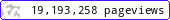•••••MadAsMaths.com :: Maths Booklets :: Further Topics :: Integration

FURTHER TOPICS - INTEGRATION

These booklets are suitable for

• students taking Further Mathematics at A level mathematics.

• undergraduate students in degrees with a significant amount of mathematics

 1st_order_differential_equations_exam_questions.pdf1st_order_differential_equations_practice.pdf1st_order_differential_equations_substitutions.pdf2nd_order_differential_equations_exam_questions.pdf2nd_order_differential_equations_practice.pdf2nd_order_differential_equations_substitutions.pdfdifferential_equations_numerical_methods.pdfarclength_surface_of_revolution.pdfimproper_integrals.pdfintegration_definite_megamix.pdfintegration_definite_megamix_student_version.pdfintegration_indefinite_megamix.pdfintegration_indefinite_megamix_student_version.pdfintegration_indefinite_megamix_student_version_condense.pdfintegration_structured_exam_questions_part_ii.pdfreduction_formulas.pdf